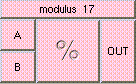MODULUSTHE BOX'S MAIN HELP: This function returns the remainder of A divided by B. A (input): This is the value to be divided. The input must be a scalar. B (input): This is the modulo value. The input must be a scalar. OUT (output): This function returns the remainder of A divided by B.

<-- BACK TO Math